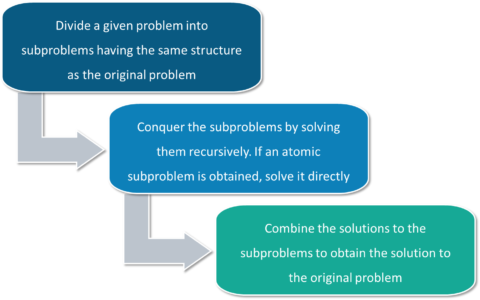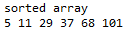# How To Implement QuickSort in Java?

Published on Aug 20,2019 798 ViewsQuickSort is a divide & conquer algorithm. In Divide & Conquer algorithm design paradigm, we divide the problems in sub-problems recursively then solve the sub-problems & at last combine the solutions to find the final result. In this article we will focus on QuickSort In Java

Lets begin!

One thing to keep in mind while dividing the problems into sub-problems is that the structure of sub-problems does not change as of the original problem.
Divide & Conquer algorithm has 3 steps:

• Divide: Breaking the problem into subproblems
• Conquer: Recursively solving the subproblems
• Combine: Combining the solutions to get the final resultThere are various algorithms based on divide & conquer paradigm. Quick sort & Merge sort are amongst them.

Although the worst-case time complexity of QuickSort is O(n2) which is more than many other sorting algorithms like Merge Sort and Heap Sort, QuickSort is faster in practice, because its inner loop can be efficiently implemented on most architectures, and in most real-world data.Let’s talk about the implementation of Quick sort algorithm. Quicksort algorithms take a pivot element and partitions the array around the pivot elememt. There are number of variations of Quicksot which depends on how you choose the pivot element. There of multiple ways to choose the pivot element:

• Picking the first element
• Pick the last element
• Picking a random element
• Picking median element

Next important thing to understand is, the partition() function in Quick sort algorithm. Partition function to take a pivot element, places it at right position, moves all the elements smaller than the pivot element to its left & all the elements greater to its right. Quicksort takes linear time to do so.
Then the array is divided in two parts from the pivot element (i.e. elements less than pivot & elements greater than pivot) & both the arrays are recursively sorted using Quicksort algorithm.Now that we understand the working of QuickSort algorithm. Let’s understand how to imlplement Quicksort algorithm in Java.

## QuickSort Function:

/* Quicksort Function needs the array to be sorted with the lowest & highest index */

```void sort(int arr[], int lowIndex, int highIndex)
{
//Until lowIndex = highIndex
if (lowIndex < highIndex)
{
// partitioning of the array
int p = partition(arr, lowIndex, highIndex);
// Recursively sort elements before & after partition
sort(arr, lowIndex, p-1);
sort(arr, p+1, highIndex);
}
}
```

Now let us look at the partitioning code to understand how it works.

## Partition Code

In the partitioning code, we will pick the last element as the pivot element. We traverse the complete array (i.e. using variable j in our case). We keep track of the last smallest element in the array (i.e. using variable i in our case). If we find any element smaller than the pivot, we move swap current element a[j] with arr[i], else we continue to traverse.

```int partition(int arr[], int lowIndex, int highIndex)
{
//Making the last element as pivot
int pivot = arr[highIndex];
//Using i to keep track of smaller elements from pivot
int i = (lowIndex-1);
for (int j=lowIndex; j<highIndex; j++)
{
// If current element is smaller than or equal to pivot
if (arr[j] <= pivot)
{
i++; // increment i
//swap ith element with jth element
int temp = arr[i];
arr[i] = arr[j];
arr[j] = temp;
}
}
// moving pivot at its correct position
int temp = arr[i+1];
arr[i+1] = arr[highIndex];
arr[highIndex] = temp;
return i+1;
}
```

Now that you have understood Quicksort & partition function, let us quick look at the complete code

## QuickSort Java Code

```class QuickSort
{
// Partition Method
int partition(int arr[], int lowIndex, int highIndex)
{
int pivot = arr[highIndex];
int i = (lowIndex-1);
for (int j=lowIndex; j<highIndex; j++)
{
if (arr[j] <= pivot)
{
i++;
int temp = arr[i];
arr[i] = arr[j];
arr[j] = temp;
}
}
int temp = arr[i+1];
arr[i+1] = arr[highIndex];
arr[highIndex] = temp;
return i+1;
}
```

// Sorting Method

```void sort(int arr[], int lowIndex, int highIndex)
{
if (lowIndex < highIndex)
{
int pi = partition(arr, lowIndex, highIndex);
sort(arr, lowIndex, pi-1);
sort(arr, pi+1, highIndex);
}
}
```

// Method to print array

```static void printArray(int arr[])
{
int n = arr.length;
for (int i=0; i<n; ++i)
System.out.print(arr[i]+" ");
System.out.println();
}
```

// Main Method

```public static void main(String args[])
{
int arr[] = {101, 37, 68, 29, 11, 5};
int n = arr.length;
QuickSort ob = new QuickSort();
ob.sort(arr, 0, n-1);
System.out.println("sorted array");
printArray(arr);
}
}
```

Output:Now after executing the above Java program you would have understood how QuickSort works & how to implement it in Java. Thus we have come to an end of this article on ‘Quicksort in Java’. If you wish to learn more, check out the Java Training by Edureka, a trusted online learning company. Edureka’s Java J2EE and SOA training and certification course is designed to train you for both core and advanced Java concepts along with various Java frameworks like Hibernate & Spring.

Got a question for us? Please mention it in the comments section of this blog  and we will get back to you as soon as possible.REGISTER FOR FREE WEBINARThank you for registering Join Edureka Meetup community for 100+ Free Webinars each month